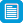# Search results

## Refine by topic

Main topic Specific topic Related topic
Listed under:  Mathematics  >  Angles### reSolve: Mechanical Linkages: Geometry Proofs

This series of six lessons explores geometry proofs using real world contexts focused on the dynamics of linkages and moving joints. Students use physical models and computer simulations, the lessons move from a view of geometry as a study to static diagrams to encompass movement. Each lesson is outlined in detail including ...### Angles - measurement, construction, estimation

This is a website designed for teachers and students in year 5, and addresses the topic of angles. It contains material on the vocabulary used when dealing with angles, and introduces the measurement and construction of angles using a protractor. There are pages for both teachers and students. The student pages contain ...### Numeracy wrap: It's what's inside that counts

interactive activities that guide students to explore the interior and exterior angle sums of polygons.### Finding Angles From Ratios

An animated tutorial about using a calculator to find an angle when given a trigonometric ratio. An interactive quiz is included.### Numeracy wrap: Tell me why

Interactive activities that guide students to consider the use and presentation of geometric reasoning.### reSolve: Authentic Problems: Pyramids in a Box

This sequence of four lessons focuses on working with solids and their nets. The lessons provide opportunities for students to work flexibly as they construct simple prisms and pyramids from nets they have created. Students record their mathematical thinking as they work through iterations to refine a box that has the least ...### Geometric reasoning - congruence

This is a website designed for both teachers and students that introduces congruence of shapes in the plane through transformations. In particular, transformations, translations, reflections in an axis and rotations of multiples of 90 degrees are used to define congruence and to identify congruent shapes. The four congruence ...### Numeracy wrap: Parallelogram peculiarities

Interactive activities that guide students to investigate properties of parallelograms.This series of six lessons explores geometry using real world contexts focussed on the dynamics of linkages and moving joints of everyday tools and objects. Students use physical models and computer simulations, the lessons move from a view of geometry as a study static diagrams to encompass movement. Each lesson is outlined ...### The geometer's warehouse

This web-based, multimedia resource focuses on the geometry of the Stage 4 and Stage 5 Mathematics syllabus. It comprises 70 dynamic html worksheets, each exploring a different outcome in Stage 4 and Stage 5 geometry.### reSolve: Mechanical Linkages: Angles and Lines

This sequence of lessons explores the geometry of angles using real world contexts including the dynamics of folding and joints. Students investigate side lengths and angles, supported by using physical models and computer simulation. There are opportunities to develop geometric language and to highlight how mathematical ...### TIMES Module 9: Measurement and Geometry: introduction to plane geometry - teacher guide

This is a 16-page guide for teachers. It provides an introduction to the initial ideas of plane geometry. Points and lines are introduced as fundamental objects in the study of geometry. Angles and parallelism are the initial areas of attention in a more formal approach to geometry that occurs from year 7.### Tessellating tiles

In this teaching resource students learn how to construct shapes that will tessellate (or tile) a plane area. Starting with a regular shape known to tessellate (square, equilateral triangle, hexagon), students apply geometrical transformations to the sides of the shape to create new shapes that tessellate. There are links ...### TIMES Module 20: Measurement and Geometry: parallelograms and rectangles - teacher guide

This is a 17-page guide for teachers. It contains the definitions, properties and tests for parallelograms and rectangles. Proofs of the properties and tests are given. Constructions for parallelograms and rectangles are given.